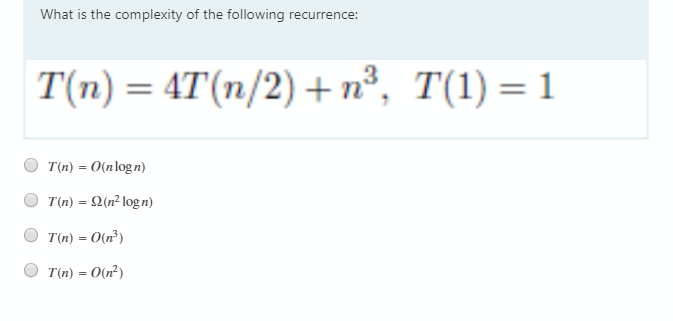# What is the complexity of the following recurrence: T(n) = 4T(n/2) + n³, T(1) = 1 T(n) = O(nlogn) T(n) = 2(n² logn) T(n) = O(n³) T(n) = O(n²)

Questionhelp_outlineImage TranscriptioncloseWhat is the complexity of the following recurrence: T(n) = 4T(n/2) + n³, T(1) = 1 T(n) = O(nlogn) T(n) = 2(n² logn) T(n) = O(n³) T(n) = O(n²) fullscreen

### Want to see this answer and more?

Experts are waiting 24/7 to provide step-by-step solutions in as fast as 30 minutes!*

*Response times vary by subject and question complexity. Median response time is 34 minutes and may be longer for new subjects.
Tagged in
EngineeringComputer Science

### Algorithms